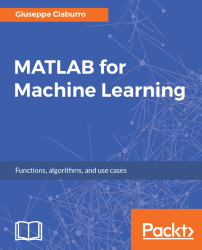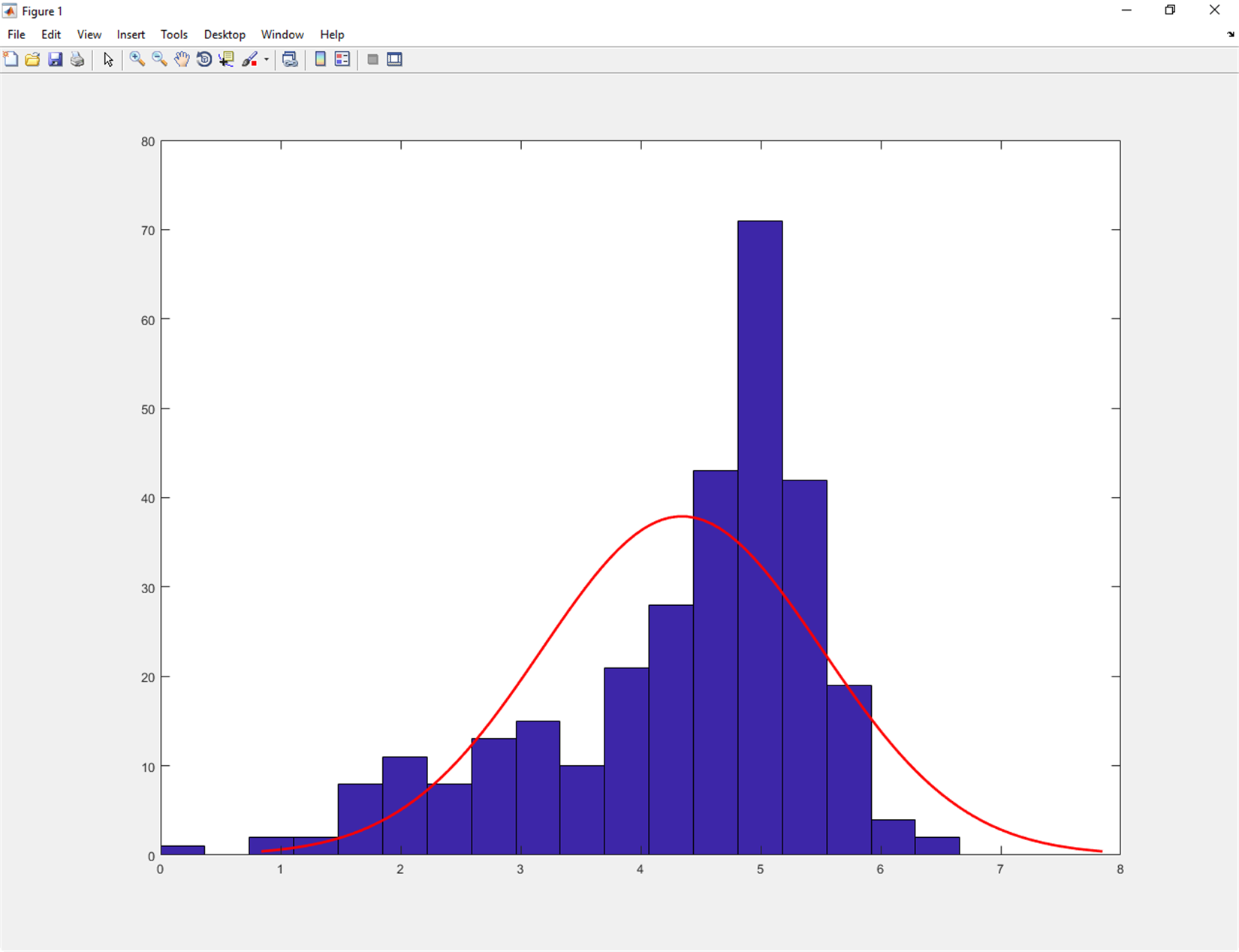•#### MATLAB for Machine Learning#### Overview of this book

MATLAB is the language of choice for many researchers and mathematics experts for machine learning. This book will help you build a foundation in machine learning using MATLAB for beginners. You’ll start by getting your system ready with t he MATLAB environment for machine learning and you’ll see how to easily interact with the Matlab workspace. We’ll then move on to data cleansing, mining and analyzing various data types in machine learning and you’ll see how to display data values on a plot. Next, you’ll get to know about the different types of regression techniques and how to apply them to your data using the MATLAB functions. You’ll understand the basic concepts of neural networks and perform data fitting, pattern recognition, and clustering analysis. Finally, you’ll explore feature selection and extraction techniques for dimensionality reduction for performance improvement. At the end of the book, you will learn to put it all together into real-world cases covering major machine learning algorithms and be comfortable in performing machine learning with MATLAB.
Title PageCreditsForewordwww.PacktPub.comCustomer FeedbackPrefaceFree Chapter
Getting Started with MATLAB Machine LearningImporting and Organizing Data in MATLABFrom Data to Knowledge DiscoveryFinding Relationships between Variables - Regression TechniquesPattern Recognition through Classification AlgorithmsIdentifying Groups of Data Using Clustering MethodsSimulation of Human Thinking - Artificial Neural NetworksImproving the Performance of the Machine Learning Model - Dimensionality ReductionMachine Learning in Practice## Statistics and algebra in MATLAB

Machine learning is an interdisciplinary field; it includes statistics, probability theory, algebra, computer science, and much more. These disciplines come together in algorithms capable of learning iteratively from data and finding hidden insights that can be used to create intelligent applications. In spite of the immense possibilities offered by machine learning, a thorough mathematical understanding of many of these disciplines is necessary for a good understanding of the inner workings of the algorithms and getting good results.

There are many reasons why the statistics and algebra is important to build a machine learning system. Some of them are highlighted as follows:

• Select the right algorithm in terms of accuracy, training time, number of parameters, number of features, and complexity of the model
• Correctly set the parameters and choose validation strategies
• Recognize underfitting and overfitting
• Put appropriate confidence interval and uncertainty

MATLAB offers several functions that allow us to perform statistical analyses and algebraic operations on our data. For example, in MATLAB, computing descriptive statistics from sample data really is a breeze. It is possible to measure central tendency, dispersion, shape, correlation, covariance, quantiles, percentiles and much more. In addition, we can tabulate and cross-tabulate data, and compute summary statistics for grouped data. In case of missing (NaN) values, MATLAB arithmetic operation functions return NaN. To solve this problem, available functions in Statistics and Machine Learning Toolbox ignore these missing values and return a numerical value calculated using the remaining values.

Furthermore we can use statistical visualization to understand how data is distributed and how that compares to other datasets and distributions. In MATLAB, we may explore single-variable distributions using univariate plots such as box plots and histograms. As well as we can discover the relationships between variables applying bivariate plots such as grouped scatter plots and bivariate histograms. We visualize the relationship between multiple variables using multivariate plots such as Andrews and glyph plots. Finally we may customize our plot by adding case names, least-squares lines, and reference curves.

As for statistical analysis, and also for linear algebra, MATLAB offers many solutions ready to use. To remind you, linear algebra is the study of linear equations and their properties and is based on numerical matrices. MATLAB offers many tools for manipulating matrices, easily understood by people who are not experts in them. MATLAB makes it easy to perform computations with vectors and matrices.Figure 1.22: Histogram of the sample data with a normal density fit

MATLAB provides several functions for:

• Matrix operations and transformations
• Linear equations
• Matrix factorization and decomposition
• Eigenvalues and eigenvectors
• Matrix analysis and vector calculus
• Normal forms and special matrices
• Matrix functions

With the help of these functions, performing linear algebra tasks in a MATLAB environment is really easy.